[Solved]: ModuleNotFoundError: No module named 'minecart'

You can solve this by installing minecart.

pip install minecart

[Solved]: ModuleNotFoundError: No module named 'frontend'

You can resolve this by installing PyMuPDF.

pip install PyMuPDF

Ordering of Batch Normalization and Dropout? | Order of Layers in the Model

Dropout is meant to block information from certain neurons completely to make sure the neurons do not co-adapt. So, the batch normalization has to be after dropout otherwise you are passing information through normalization statistics.

If you think about it, in typical ML problems, this is the reason we don’t compute mean and standard deviation over entire data and then split it into train, test and validation sets. We split and then compute the statistics over the train set and use them to normalize and centre the validation and test datasets

so I suggest Scheme 1

-> CONV/FC -> ReLu(or other activation) -> Dropout -> BatchNorm -> CONV/FC

as opposed to Scheme 2

-> CONV/FC -> BatchNorm -> ReLu(or other activation) -> Dropout -> CONV/FC

Please note that this means that the network under Scheme 2 should show over-fitting as compared to network under Scheme 1 but OP ran some tests as mentioned in question and they support Scheme 2

How To Calculate The Number Of Parameters For The CNN (Convolutional Neural Network)?

Let’s first look at how the number of learnable parameters is calculated for each individual type of layer you have, and then calculate the number of parameters in your example.

• Input layer: All the input layer does is read the input image, so there are no parameters you could learn here.
• Convolutional layers: Consider a convolutional layer which takes l feature maps at the input, and has k feature maps as output. The filter size is n x m. For example, this will look like this: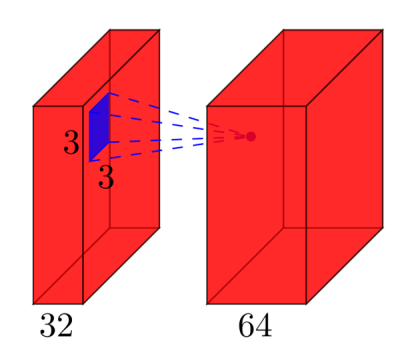Here, the input has l=32 feature maps as input, k=64 feature maps as output, and the filter size is n=3 x m=3. It is important to understand, that we don’t simply have a 3×3 filter, but actually a 3x3x32 filter, as our input has 32 dimensions. And we learn 64 different 3x3x32 filters. Thus, the total number of weights is n*m*k*l. Then, there is also a bias term for each feature map, so we have a total number of parameters of (n*m*l+1)*k.

• Pooling layers: The pooling layers e.g. do the following: “replace a 2×2 neighborhood by its maximum value”. So there is no parameter you could learn in a pooling layer.
• Fully-connected layers: In a fully-connected layer, all input units have a separate weight to each output unit. For n inputs and m outputs, the number of weights is n*m. Additionally, you have a bias for each output node, so you are at (n+1)*m parameters.
• Output layer: The output layer is a normal fully-connected layer, so (n+1)*m parameters, where n is the number of inputs and m is the number of outputs.

The final difficulty is the first fully-connected layer: we do not know the dimensionality of the input to that layer, as it is a convolutional layer. To calculate it, we have to start with the size of the input image, and calculate the size of each convolutional layer. In your case, Lasagne already calculates this for you and reports the sizes – which makes it easy for us. If you have to calculate the size of each layer yourself, it’s a bit more complicated:

• In the simplest case (like your example), the size of the output of a convolutional layer is input_size - (filter_size - 1), in your case: 28 – 4 = 24. This is due to the nature of the convolution: we use e.g. a 5×5 neighborhood to calculate a point – but the two outermost rows and columns don’t have a 5×5 neighborhood, so we can’t calculate any output for those points. This is why our output is 2*2=4 rows/columns smaller than the input.
• If one doesn’t want the output to be smaller than the input, one can zero-pad the image (with the pad parameter of the convolutional layer in Lasagne). E.g. if you add 2 rows/cols of zeros around the image, the output size will be (28+4)-4=28. So in case of padding, the output size is input_size + 2*padding - (filter_size -1).
• If you explicitly want to downsample your image during the convolution, you can define a stride, e.g. stride=2, which means that you move the filter in steps of 2 pixels. Then, the expression becomes ((input_size + 2*padding - filter_size)/stride) +1.

In your case, the full calculations are:

#  name                           size                 parameters---  --------  -------------------------    ------------------------  0  input                       1x28x28                           0  1  conv2d1   (28-(5-1))=24 -> 32x24x24    (5*5*1+1)*32   =     832  2  maxpool1                   32x12x12                           0  3  conv2d2   (12-(3-1))=10 -> 32x10x10    (3*3*32+1)*32  =   9'248  4  maxpool2                     32x5x5                           0  5  dense                           256    (32*5*5+1)*256 = 205'056  6  output                           10    (256+1)*10     =   2'570

So in your network, you have a total of 832 + 9’248 + 205’056 + 2’570 = 217’706 learnable parameters, which is exactly what Lasagne reports.

How to verify CuDNN installation?

Installing CuDNN just involves placing the files in the CUDA directory. If you have specified the routes and the CuDNN option correctly while installing caffe it will be compiled with CuDNN.

You can check that using cmake. Create a directory caffe/build and run cmake .. from there. If the configuration is correct you will see these lines:

-- Found cuDNN (include: /usr/local/cuda-7.0/include, library: /usr/local/cuda-7.0/lib64/libcudnn.so)-- NVIDIA CUDA:--   Target GPU(s)     :   Auto--   GPU arch(s)       :   sm_30--   cuDNN             :   Yes

If everything is correct just run the make orders to install caffe from there.

How To Calculate A Net's Flops In CNN

if you use Keras and TensorFlow as Backend then you can try the following example. It calculates the FLOPs for the MobileNet.

import tensorflow as tfimport keras.backend as Kfrom keras.applications.mobilenet import MobileNetrun_meta = tf.RunMetadata()with tf.Session(graph=tf.Graph()) as sess:    K.set_session(sess)    net = MobileNet(alpha=.75, input_tensor=tf.placeholder('float32', shape=(1,32,32,3)))    opts = tf.profiler.ProfileOptionBuilder.float_operation()        flops = tf.profiler.profile(sess.graph, run_meta=run_meta, cmd='op', options=opts)    opts = tf.profiler.ProfileOptionBuilder.trainable_variables_parameter()        params = tf.profiler.profile(sess.graph, run_meta=run_meta, cmd='op', options=opts)    print("{:,} --- {:,}".format(flops.total_float_ops, params.total_parameters))

Custom Loss Function Keras Python | How to create a custom loss function in Keras

All you have to do is define a function for that, using keras backend functions for calculations. The function must take the true values and the model predicted values.

Now, since I’m not sure about what are g, q, x an y in your function, I’ll just create a basic example here without caring about what it means or whether it’s an actual useful function:

import keras.backend as Kdef customLoss(yTrue,yPred):    return K.sum(K.log(yTrue) - K.log(yPred))

All backend functions can be seen here: https://keras.io/backend/#backend-functions

After that, compile your model using that function instead of a regular one:

model.compile(loss=customLoss, optimizer = .....)

1d 2d and 3d Convolution in CNN | CNN 1D vs 2D vs 3D

I want to explain with picture from C3D.

In a nutshell, convolutional direction & output shape is important!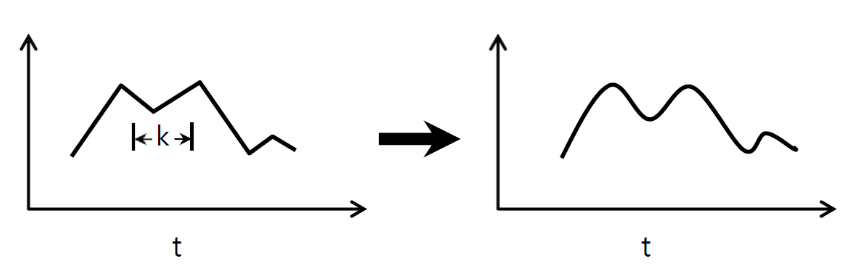↑↑↑↑↑ 1D Convolutions – Basic ↑↑↑↑↑

• just 1-direction (time-axis) to calculate conv
• input = [W], filter = [k], output = [W]
• ex) input = [1,1,1,1,1], filter = [0.25,0.5,0.25], output = [1,1,1,1,1]
• output-shape is 1D array
• example) graph smoothing

tf.nn.conv1d code Toy Example

import tensorflow as tfimport numpy as npsess = tf.Session()ones_1d = np.ones(5)weight_1d = np.ones(3)strides_1d = 1in_1d = tf.constant(ones_1d, dtype=tf.float32)filter_1d = tf.constant(weight_1d, dtype=tf.float32)in_width = int(in_1d.shape)filter_width = int(filter_1d.shape)input_1d   = tf.reshape(in_1d, [1, in_width, 1])kernel_1d = tf.reshape(filter_1d, [filter_width, 1, 1])output_1d = tf.squeeze(tf.nn.conv1d(input_1d, kernel_1d, strides_1d, padding='SAME'))print sess.run(output_1d)↑↑↑↑↑ 2D Convolutions – Basic ↑↑↑↑↑

• 2-direction (x,y) to calculate conv
• output-shape is 2D Matrix
• input = [W, H], filter = [k,k] output = [W,H]
• example) Sobel Egde Fllter

tf.nn.conv2d – Toy Example

ones_2d = np.ones((5,5))weight_2d = np.ones((3,3))strides_2d = [1, 1, 1, 1]in_2d = tf.constant(ones_2d, dtype=tf.float32)filter_2d = tf.constant(weight_2d, dtype=tf.float32)in_width = int(in_2d.shape)in_height = int(in_2d.shape)filter_width = int(filter_2d.shape)filter_height = int(filter_2d.shape)input_2d   = tf.reshape(in_2d, [1, in_height, in_width, 1])kernel_2d = tf.reshape(filter_2d, [filter_height, filter_width, 1, 1])output_2d = tf.squeeze(tf.nn.conv2d(input_2d, kernel_2d, strides=strides_2d, padding='SAME'))print sess.run(output_2d)↑↑↑↑↑ 3D Convolutions – Basic ↑↑↑↑↑

• 3-direction (x,y,z) to calcuate conv
• output-shape is 3D Volume
• input = [W,H,L], filter = [k,k,d] output = [W,H,M]
• d < L is important! for making volume output
• example) C3D

tf.nn.conv3d – Toy Example

ones_3d = np.ones((5,5,5))weight_3d = np.ones((3,3,3))strides_3d = [1, 1, 1, 1, 1]in_3d = tf.constant(ones_3d, dtype=tf.float32)filter_3d = tf.constant(weight_3d, dtype=tf.float32)in_width = int(in_3d.shape)in_height = int(in_3d.shape)in_depth = int(in_3d.shape)filter_width = int(filter_3d.shape)filter_height = int(filter_3d.shape)filter_depth = int(filter_3d.shape)input_3d   = tf.reshape(in_3d, [1, in_depth, in_height, in_depth, 1])kernel_3d = tf.reshape(filter_3d, [filter_depth, filter_height, filter_width, 1, 1])output_3d = tf.squeeze(tf.nn.conv3d(input_3d, kernel_3d, strides=strides_3d, padding='SAME'))print sess.run(output_3d)↑↑↑↑↑ 2D Convolutions with 3D input – LeNet, VGG, …, ↑↑↑↑↑

• Eventhough input is 3D ex) 224x224x3, 112x112x32
• output-shape is not 3D Volume, but 2D Matrix
• because filter depth = L must be matched with input channels = L
• 2-direction (x,y) to calcuate conv! not 3D
• input = [W,H,L], filter = [k,k,L] output = [W,H]
• output-shape is 2D Matrix
• what if we want to train N filters (N is number of filters)
• then output shape is (stacked 2D) 3D = 2D x N matrix.

conv2d – LeNet, VGG, … for 1 filter

in_channels = 32 # 3 for RGB, 32, 64, 128, ... ones_3d = np.ones((5,5,in_channels)) # input is 3d, in_channels = 32# filter must have 3d-shpae with in_channelsweight_3d = np.ones((3,3,in_channels)) strides_2d = [1, 1, 1, 1]in_3d = tf.constant(ones_3d, dtype=tf.float32)filter_3d = tf.constant(weight_3d, dtype=tf.float32)in_width = int(in_3d.shape)in_height = int(in_3d.shape)filter_width = int(filter_3d.shape)filter_height = int(filter_3d.shape)input_3d   = tf.reshape(in_3d, [1, in_height, in_width, in_channels])kernel_3d = tf.reshape(filter_3d, [filter_height, filter_width, in_channels, 1])output_2d = tf.squeeze(tf.nn.conv2d(input_3d, kernel_3d, strides=strides_2d, padding='SAME'))print sess.run(output_2d)

conv2d – LeNet, VGG, … for N filters

in_channels = 32 # 3 for RGB, 32, 64, 128, ... out_channels = 64 # 128, 256, ...ones_3d = np.ones((5,5,in_channels)) # input is 3d, in_channels = 32# filter must have 3d-shpae x number of filters = 4Dweight_4d = np.ones((3,3,in_channels, out_channels))strides_2d = [1, 1, 1, 1]in_3d = tf.constant(ones_3d, dtype=tf.float32)filter_4d = tf.constant(weight_4d, dtype=tf.float32)in_width = int(in_3d.shape)in_height = int(in_3d.shape)filter_width = int(filter_4d.shape)filter_height = int(filter_4d.shape)input_3d   = tf.reshape(in_3d, [1, in_height, in_width, in_channels])kernel_4d = tf.reshape(filter_4d, [filter_height, filter_width, in_channels, out_channels])#output stacked shape is 3D = 2D x N matrixoutput_3d = tf.nn.conv2d(input_3d, kernel_4d, strides=strides_2d, padding='SAME')print sess.run(output_3d)

↑↑↑↑↑ Bonus 1×1 conv in CNN – GoogLeNet, …, ↑↑↑↑↑

• 1×1 conv is confusing when you think this as 2D image filter like sobel
• for 1×1 conv in CNN, input is 3D shape as above picture.
• it calculate depth-wise filtering
• input = [W,H,L], filter = [1,1,L] output = [W,H]
• output stacked shape is 3D = 2D x N matrix.

tf.nn.conv2d – special case 1×1 conv

in_channels = 32 # 3 for RGB, 32, 64, 128, ... out_channels = 64 # 128, 256, ...ones_3d = np.ones((1,1,in_channels)) # input is 3d, in_channels = 32# filter must have 3d-shpae x number of filters = 4Dweight_4d = np.ones((3,3,in_channels, out_channels))strides_2d = [1, 1, 1, 1]in_3d = tf.constant(ones_3d, dtype=tf.float32)filter_4d = tf.constant(weight_4d, dtype=tf.float32)in_width = int(in_3d.shape)in_height = int(in_3d.shape)filter_width = int(filter_4d.shape)filter_height = int(filter_4d.shape)input_3d   = tf.reshape(in_3d, [1, in_height, in_width, in_channels])kernel_4d = tf.reshape(filter_4d, [filter_height, filter_width, in_channels, out_channels])#output stacked shape is 3D = 2D x N matrixoutput_3d = tf.nn.conv2d(input_3d, kernel_4d, strides=strides_2d, padding='SAME')print sess.run(output_3d)

Animation (2D Conv with 3D-inputs)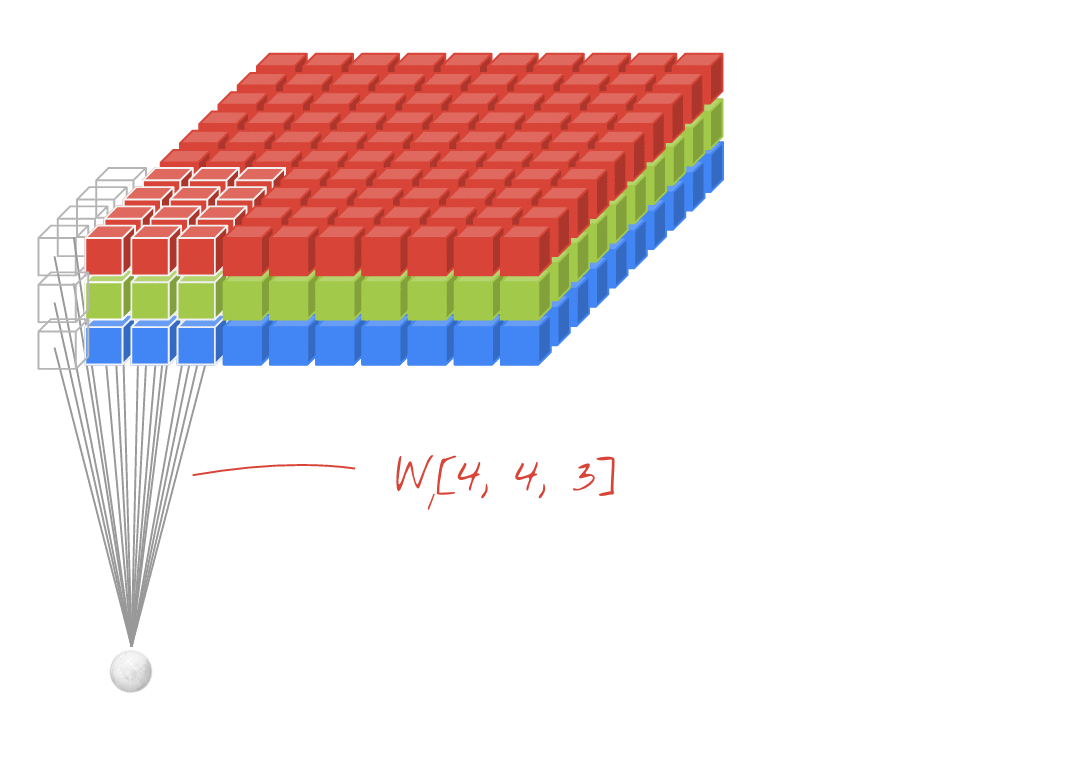– Original Link : LINK
– The author: Martin Görner

Bonus 1D Convolutions with 2D input↑↑↑↑↑ 1D Convolutions with 1D input ↑↑↑↑↑↑↑↑↑↑ 1D Convolutions with 2D input ↑↑↑↑↑

• Eventhough input is 2D ex) 20×14
• output-shape is not 2D , but 1D Matrix
• because filter height = L must be matched with input height = L
• 1-direction (x) to calcuate conv! not 2D
• input = [W,L], filter = [k,L] output = [W]
• output-shape is 1D Matrix
• what if we want to train N filters (N is number of filters)
• then output shape is (stacked 1D) 2D = 1D x N matrix.

Bonus C3D

in_channels = 32 # 3, 32, 64, 128, ... out_channels = 64 # 3, 32, 64, 128, ... ones_4d = np.ones((5,5,5,in_channels))weight_5d = np.ones((3,3,3,in_channels,out_channels))strides_3d = [1, 1, 1, 1, 1]in_4d = tf.constant(ones_4d, dtype=tf.float32)filter_5d = tf.constant(weight_5d, dtype=tf.float32)in_width = int(in_4d.shape)in_height = int(in_4d.shape)in_depth = int(in_4d.shape)filter_width = int(filter_5d.shape)filter_height = int(filter_5d.shape)filter_depth = int(filter_5d.shape)input_4d   = tf.reshape(in_4d, [1, in_depth, in_height, in_depth, in_channels])kernel_5d = tf.reshape(filter_5d, [filter_depth, filter_height, filter_width, in_channels, out_channels])output_4d = tf.nn.conv3d(input_4d, kernel_5d, strides=strides_3d, padding='SAME')print sess.run(output_4d)sess.close()

Input & Output in Tensorflow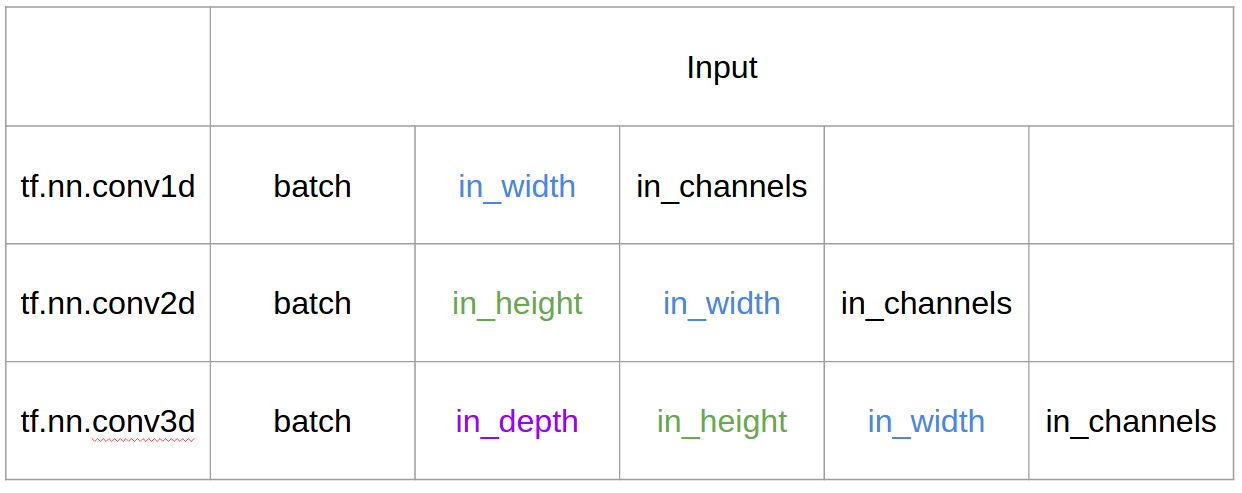Summary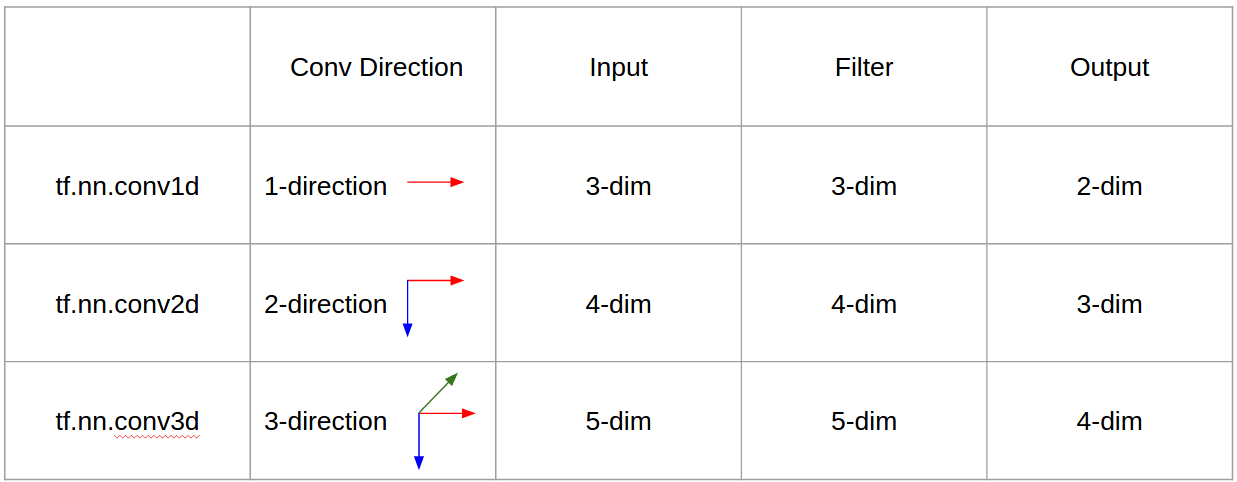Batch Normalization in CNN

Let’s start with the terms. Remember that the output of the convolutional layer is a 4-rank tensor [B, H, W, C], where B is the batch size, (H, W) is the feature map size, C is the number of channels. An index (x, y) where 0 <= x < H and 0 <= y < W is a spatial location.

Usual batchnorm

Now, here’s how the batchnorm is applied in a usual way (in pseudo-code):

# t is the incoming tensor of shape [B, H, W, C]# mean and stddev are computed along 0 axis and have shape [H, W, C]mean = mean(t, axis=0)stddev = stddev(t, axis=0)for i in 0..B-1:  out[i,:,:,:] = norm(t[i,:,:,:], mean, stddev)

Basically, it computes H*W*C means and H*W*C standard deviations across B elements. You may notice that different elements at different spatial locations have their own mean and variance and gather only B values.

Batchnorm in conv layer

This way is totally possible. But the convolutional layer has a special property: filter weights are shared across the input image (you can read it in detail in this post). That’s why it’s reasonable to normalize the output in the same way, so that each output value takes the mean and variance of B*H*W values, at different locations.

Here’s how the code looks like in this case (again pseudo-code):

# t is still the incoming tensor of shape [B, H, W, C]# but mean and stddev are computed along (0, 1, 2) axes and have just [C] shapemean = mean(t, axis=(0, 1, 2))stddev = stddev(t, axis=(0, 1, 2))for i in 0..B-1, x in 0..H-1, y in 0..W-1:  out[i,x,y,:] = norm(t[i,x,y,:], mean, stddev)

In total, there are only C means and standard deviations and each one of them is computed over B*H*W values. That’s what they mean when they say “effective mini-batch”: the difference between the two is only in axis selection (or equivalently “mini-batch selection”).

Different Ways to Do Batch-norm in Tensorflow

there are several more ways to do batch-norm in TensorFlow:

• tf.nn.batch_normalization is a low-level op. The caller is responsible to handle mean and variance tensors themselves.
• tf.nn.fused_batch_norm is another low-level op, similar to the previous one. The difference is that it’s optimized for 4D input tensors, which is the usual case in convolutional neural networks. tf.nn.batch_normalization accepts tensors of any rank greater than 1.
• tf.layers.batch_normalization is a high-level wrapper over the previous ops. The biggest difference is that it takes care of creating and managing the running mean and variance tensors, and calls a fast fused op when possible. Usually, this should be the default choice for you.
• tf.contrib.layers.batch_norm is the early implementation of batch norm, before it’s graduated to the core API (i.e., tf.layers). The use of it is not recommended because it may be dropped in the future releases.
• tf.nn.batch_norm_with_global_normalization is another deprecated op. Currently, delegates the call to tf.nn.batch_normalization, but likely to be dropped in the future.
• Finally, there’s also Keras layer keras.layers.BatchNormalization, which in case of tensorflow backend invokes tf.nn.batch_normalization.

Where to add BatchNormalization function in Keras?|Batch Normalization Keras Example

Batch Normalization is just another layer, so you can use it as such to create your desired network architecture.

The general use case is to use BN between the linear and non-linear layers in your network, because it normalizes the input to your activation function, so that you’re centered in the linear section of the activation function (such as Sigmoid). There’s a small discussion of it here

In your case above, this might look like:

Paragraph Detection In Image Python Using OpenCV

This a classic use for cv2.dilate(). Essentially when you want to connect items together, you can dilate them to join multiple items into a single item. Here’s a simple approach

• Convert image to grayscale and Gaussian Blur
• Dilate to connect adjacent words together
• Find contours and draw bounding box
import cv2import numpy as npimage = cv2.imread('test.png')gray = cv2.cvtColor(image, cv2.COLOR_BGR2GRAY)blur = cv2.GaussianBlur(gray, (7,7), 0)thresh = cv2.threshold(blur, 0, 255, cv2.THRESH_BINARY_INV + cv2.THRESH_OTSU)kernel = cv2.getStructuringElement(cv2.MORPH_RECT, (5,5))dilate = cv2.dilate(thresh, kernel, iterations=4)cnts = cv2.findContours(dilate, cv2.RETR_EXTERNAL, cv2.CHAIN_APPROX_SIMPLE)cnts = cnts if len(cnts) == 2 else cntsfor c in cnts:    x,y,w,h = cv2.boundingRect(c)    cv2.rectangle(image, (x, y), (x + w, y + h), (36,255,12), 2)cv2.imshow('th', thresh)cv2.imshow('dilated', dilate)cv2.imshow('image', image)cv2.waitKey()

Here’s where the magic happens. We can assume that a paragraph is a section of words that are close together, to achieve this we dilate to connect adjacent words.[Solved]: How To Split A String Into Multiple Lines In YAML?

1. Block Notation(plain, flow-style, scalar): Newlines become spaces and extra newlines after the block are removed

---# Note: It has 1 new line after the stringcontent:    Arbitrary free text    over multiple lines stopping    after indentation changes......

Equivalent JSON

{ "content": "Arbitrary free text over multiple lines stopping after indentation changes..."}

2. Literal Block Scalar: A Literal Block Scalar | will include the newlines and any trailing spaces. but removes extra

newlines after the block.

---# After string we have 2 spaces and 2 new linescontent1: | Arbitrary free text over "multiple lines" stopping after indentation changes...  ...

Equivalent JSON

{ "content1": "Arbitrary free textnover "multiple lines" stoppingnafter indentation changes...  n"}

3. + indicator with Literal Block Scalar: keep extra newlines after block

---# After string we have 2 new linesplain: |+ This unquoted scalar spans many lines....

Equivalent JSON

{ "plain": "This unquoted scalarnspans many lines.nnn"}

4. – indicator with Literal Block Scalar:  means that the newline at the end of the string is removed.

---# After string we have 2 new linesplain: |- This unquoted scalar spans many lines....

Equivalent JSON

{ "plain": "This unquoted scalarnspans many lines."}

5. Folded Block Scalar(>):

will fold newlines to spaces and but removes extra newlines after the block.

---folded_newlines: > this is really a single line of text despite appearances...

Equivalent JSON

{ "fold_newlines": "this is really a single line of text despite appearancesn"}

[Solved]: No module named 'imutils' after pip install

It looks like you pip installed imutils using python2’s pip and so that’s why python3 can’t import it. You might try: sudo pip3 install imutils

Python 2:

sudo pip install imutils

Python 3:

sudo pip3 install imutils

Even after installing if it is showing error then use this :

How to Add a Blank Page in the Existing PDF in Java?

Answer: You can use PdfStamper for this purpose.